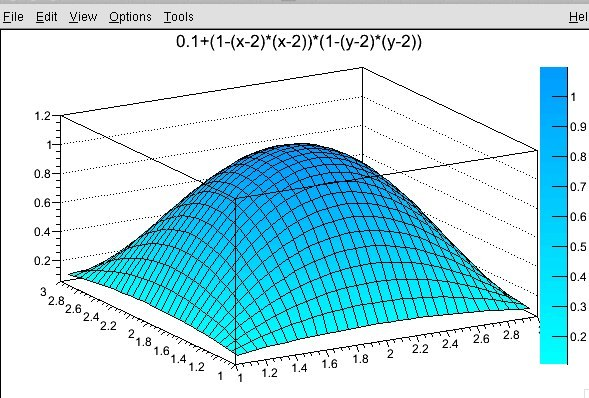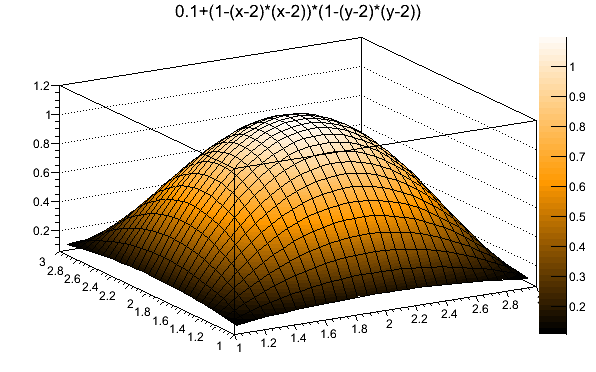I would like to use TColor::CreateGradientColorTable() to make a color palette that goes smoothly from black to orange to white. I use the example given in the documentation, where I change the rgb values to correspond to the colors I want at the stop, black, orange, white.

``````{
TCanvas *c2  = new TCanvas("c2","c2",0,0,600,400);
TF2 *f2 = new TF2("f2","0.1+(1-(x-2)*(x-2))*(1-(y-2)*(y-2))",1,3,1,3);
UInt_t Number = 3;
Double_t Red[Number]    = { 0.00, 0.00, 0.00};
Double_t Green[Number]  = { 1.00, 0.60, 0.00};
Double_t Blue[Number]   = { 1.00, 1.00, 1.00};
Double_t Length[Number] = { 0.00, 0.5, 1.00 };
Int_t nb=50;
f2->SetContour(nb);
f2->Draw("surf1z");
return c2;
}``````

In the output, I get shades of blue (see below). I experimented with other values, but somehow, they do not make sense. How can I get the colors I need?You miss-defined the parameters. It should be:

``````{
TCanvas *c2  = new TCanvas("c2","c2",0,0,600,400);
TF2 *f2 = new TF2("f2","0.1+(1-(x-2)*(x-2))*(1-(y-2)*(y-2))",1,3,1,3);
UInt_t Number = 3;
Double_t Red[Number]    = { 0.00, 1.00, 1.00};
Double_t Green[Number]  = { 0.00, 0.60, 1.00};
Double_t Blue[Number]   = { 0.00, 0.00, 1.00};
Double_t Length[Number] = { 0.00, 0.50, 1.00 };
Int_t nb=50;
f2->SetContour(nb);
f2->Draw("surf1z");
return c2;
}``````Thank you for the response. Could you explain how you got the RGB values? These seem to be shades of blue or am I not interpreting the arrays correctly?

Also, what does one input if one wants to use more than 3 stops since there are only three RGB arrays defined?

I took your values !!! you simply put them in the wrong way … horizontally instead of vertically …
Look closely at the code you will understand …

I think I understand now. The same index of each of the three arrays represents the RGB values for each stop you define. I assume that if you wanted, for example 4 stops, the size of the the Red, Green, and Blue arrays would be 4 instead of three.

You got it